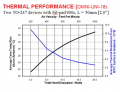# how to deduce thermal resistance of heatsinks from thermal performance graphs ?

#### Younes Thabet

Joined Jan 9, 2019
142
Hello all,

I chose this heatsink ( OMNI-UNI-18-50 ) to be used for each 2 SiC MOSFETs out of 6, I calculated the largest permissible thermal impedance between the junction and ambient air to be 4 C/W and the total power to be dissipated for every 2 MOSFETs is 36W.
The MOSFET junction-to-case thermal resistance is 0.5 C/W and the case-to-sink = 0.25 C/W (from datasheet).

Now I need the heatsink thermal resistance to be less than (4-0.5-0.25=3.25 C/W) ...
The problem is I couldn't figure out how to extract the thermal resistance of the heatsink from the graph in the datasheet!
If someone could point out how I could do that? and also check if my calculation is right.#### wayneh

Joined Sep 9, 2010
17,405
Does the right-hand axis not tell you what you need?

#### Younes Thabet

Joined Jan 9, 2019
142
Ok, got it.
I have to use total heat dissipated axis and average case temp rise above ambient axis according to EEVblog.
Although 36 watts isn't on the watts axis it gives an idea of where it would be on the graph when you follow the trajectory of the black curve and then project on the y-axis to get the temperature. it should be somewhere between 35 and 36 C.
So 36/36= 1C/W and this is assuming the heat will be distributed evenly on the surface of the heatsink but that is never the case so as a rule of thumb you increase the thermal resistance you got from the graph by a third.
So t(sink-to-ambient) = 1 +0.33 = 1.33 C/W.

#### Younes Thabet

Joined Jan 9, 2019
142
Does the right-hand axis not tell you what you need?
Actually, the left and bottom axis are the ones to look for.
I think the left and top ones represent the change in heatsink surface-to-ambient thermal resistance by airflow (in case you decided to add a DC fan for your heatsink)

#### BobTPH

Joined Jun 5, 2013
7,519
What that graph tells me is that you need a fan moving about 900 ft^3 of air per minute to achieve the thermal resistance you need.